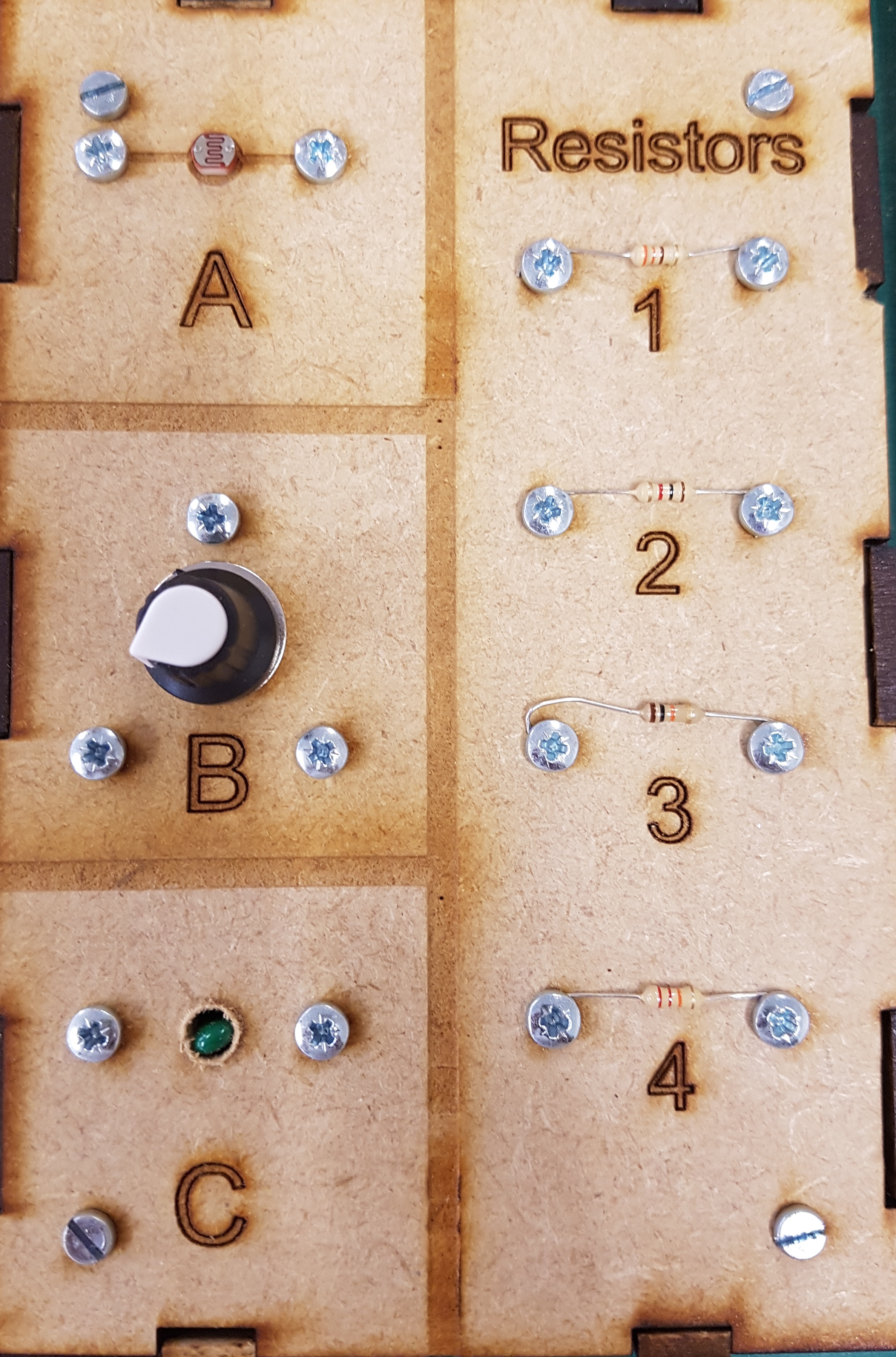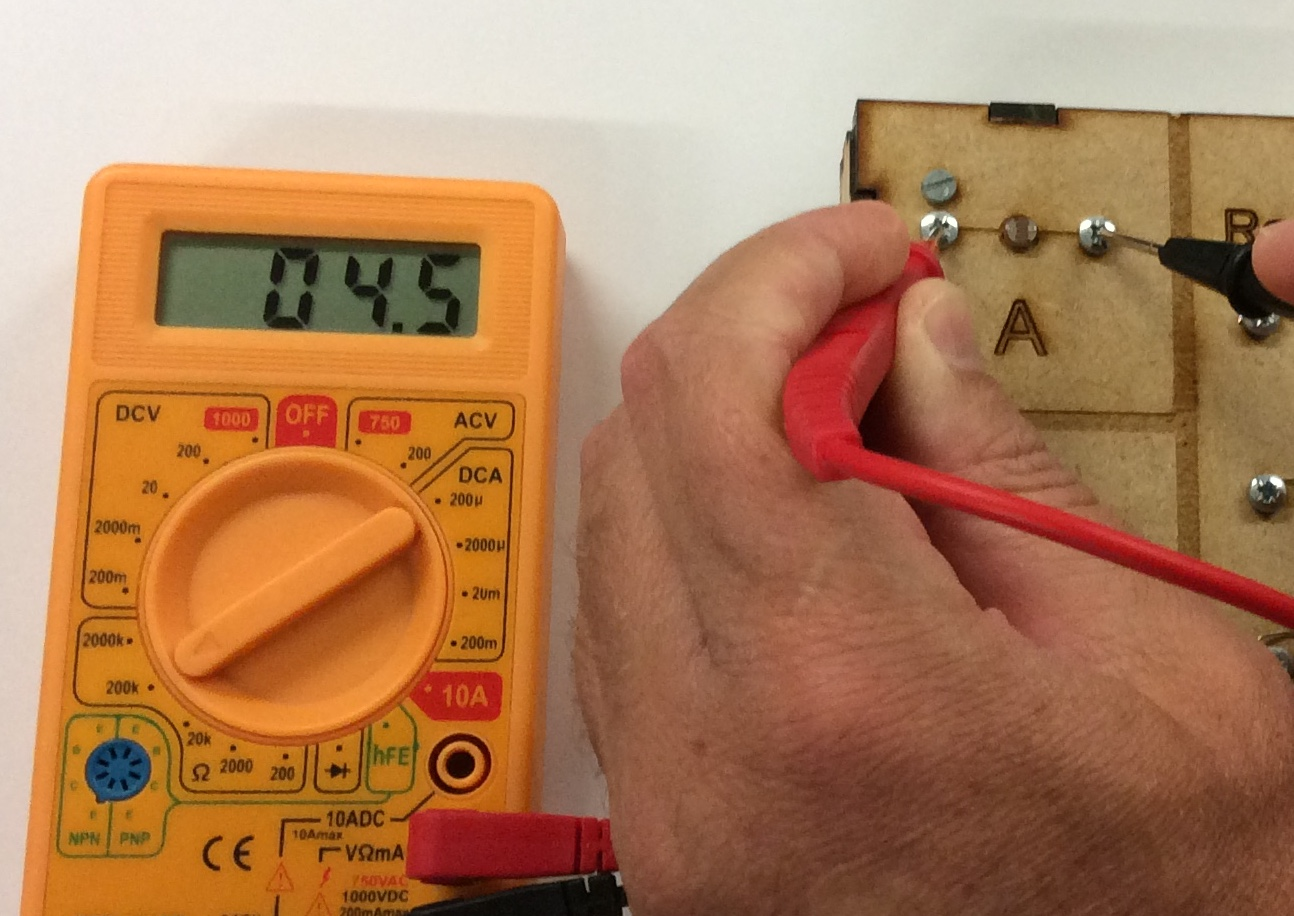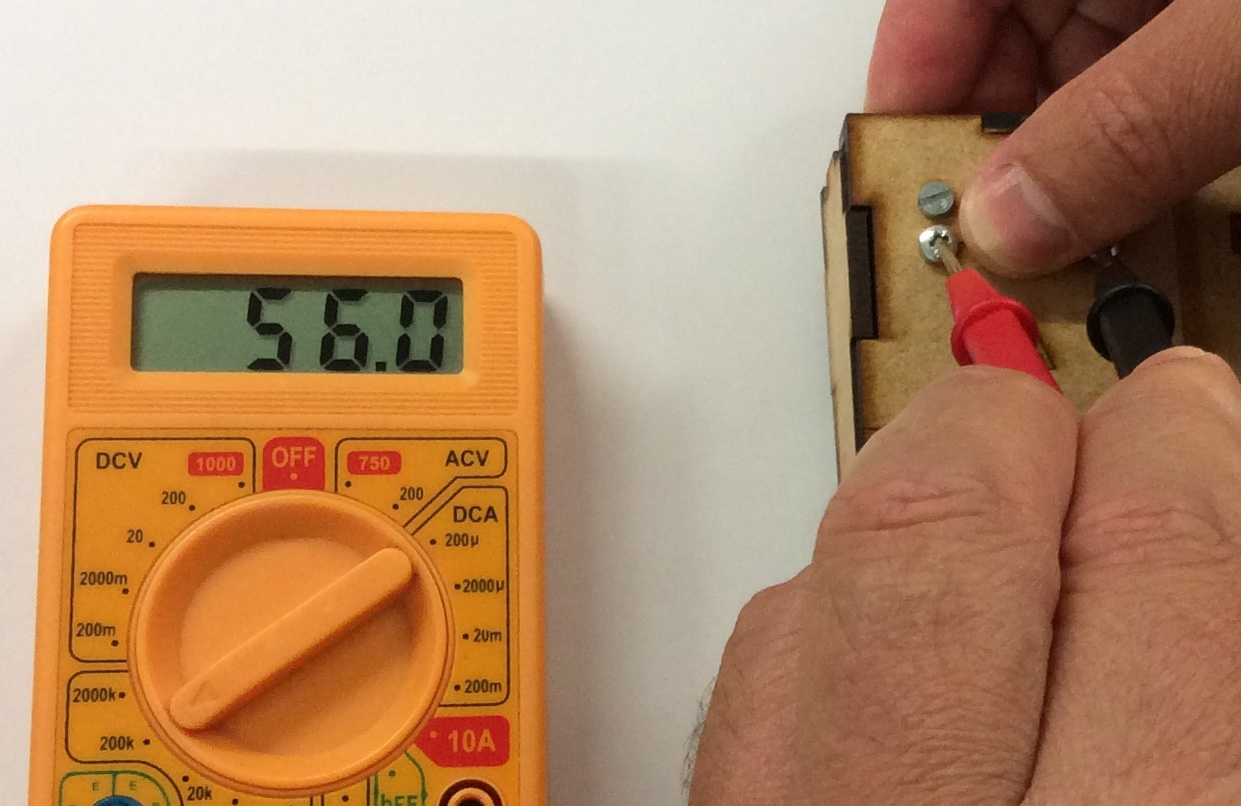# The Puzzle Boxes• Below is a video on how to use a multimeter. Please watch this before continuing testing below.

• Area A: An LDR. LDRs typically have a resistance value from 400 to 2M (2 million) Ohms, depending on the amount they are exposed to: More light = less resistance.

i. Select the 200k setting on the multimeter for Box A, B and C below. Read the resistance across the LDR, and write it down.ii. Put your finger over the LDR and read the resistance again.• Area B: The component in here is called a potentiometer. By turning the dial, the resistance between its 3 pins can be changed.

i. What is the resistance between pins 1 and 3?

ii. Turn the dial to face pin 1. What is the resistance between pins 1 and 2? What is the resistance between 2 and 3? What number do you get when these values are added together?

iii. Turn the dial to face pin 2. What is the resistance between pins 1 and 2? What is the resistance between 2 and 3? What number do you get when these values are added together?

iv. Turn the dial to face pin 3. What is the resistance between pins 1 and 2? What is the resistance between 2 and 3? What number do you get when these values are added together?
• Area C: The green component is called a thermistor(Thermal Resistor). Its resistance changes in response to temperature.

i. To test the component, you can put your finger on it and this will raise the temperature slowly. If you keep the meter on it, you should see the resistance change.

• Resistors There are 4 resistors included in the box, use a multi-meter to record the values of each resistor in the same text file you created.